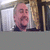## vlookupTags:
Excel 2003 VLOOKUP function
Microsoft Excel 2003
VLOOKUP function
how can I convert then match two lists of numbers?   One list has thousands of 7 digit numbers.   The other list is even longer, but contains all of the numbers in the first list, looking at the first 7 digits of each.   The difference is, the numbers have an additional digit on the end.

Software/Hardware used:
Excel 2003

Thanks. We'll let you know when a new response is added.

## Discuss This Question: 3 Replies

••